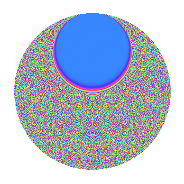# Properties

 Label 4.34.a.aLevel 4 Weight 34 Character orbit 4.a Self dual Yes Analytic conductor 27.593 Analytic rank 0 Dimension 3 CM No Inner twists 1

# Related objects

## Newspace parameters

 Level: $$N$$ = $$4 = 2^{2}$$ Weight: $$k$$ = $$34$$ Character orbit: $$[\chi]$$ = 4.a (trivial)

## Newform invariants

 Self dual: Yes Analytic conductor: $$27.5931315524$$ Analytic rank: $$0$$ Dimension: $$3$$ Coefficient field: $$\mathbb{Q}[x]/(x^{3} - \cdots)$$ Coefficient ring: $$\Z[a_1, \ldots, a_{5}]$$ Coefficient ring index: $$2^{22}\cdot 3^{3}\cdot 7\cdot 11\cdot 29$$ Fricke sign: $$-1$$ Sato-Tate group: $\mathrm{SU}(2)$

## $q$-expansion

Coefficients of the $$q$$-expansion are expressed in terms of a basis $$1,\beta_1,\beta_2$$ for the coefficient ring described below. We also show the integral $$q$$-expansion of the trace form.

 $$f(q)$$ $$=$$ $$q + ( 30830596 + \beta_{1} ) q^{3} + ( -17960227962 - 1535 \beta_{1} + \beta_{2} ) q^{5} + ( 1513669971464 - 122346 \beta_{1} + 180 \beta_{2} ) q^{7} + ( 2010788144563341 + 75248670 \beta_{1} - 10530 \beta_{2} ) q^{9} +O(q^{10})$$ $$q +(30830596 + \beta_{1}) q^{3} +(-17960227962 - 1535 \beta_{1} + \beta_{2}) q^{5} +(1513669971464 - 122346 \beta_{1} + 180 \beta_{2}) q^{7} +(2010788144563341 + 75248670 \beta_{1} - 10530 \beta_{2}) q^{9} +(75872552434149900 + 2215839115 \beta_{1} + 256840 \beta_{2}) q^{11} +(90990147405786254 + 6383188809 \beta_{1} - 3668535 \beta_{2}) q^{13} +(-10714157827080368616 - 382917087630 \beta_{1} + 33406668 \beta_{2}) q^{15} +(31012396103938905618 + 1069301430158 \beta_{1} - 184208050 \beta_{2}) q^{17} +($$$$44\!\cdots\!76$$$$+ 11850406238205 \beta_{1} + 320516280 \beta_{2}) q^{19} +(-$$$$76\!\cdots\!84$$$$- 57340224604180 \beta_{1} + 4392064620 \beta_{2}) q^{21} +($$$$38\!\cdots\!32$$$$- 94999163347438 \beta_{1} - 49295676820 \beta_{2}) q^{23} +($$$$86\!\cdots\!07$$$$+ 615284668657260 \beta_{1} + 277757168364 \beta_{2}) q^{25} +($$$$38\!\cdots\!08$$$$+ 2919170542770738 \beta_{1} - 973938527640 \beta_{2}) q^{27} +($$$$96\!\cdots\!26$$$$- 17291424904695515 \beta_{1} + 1814914414885 \beta_{2}) q^{29} +($$$$52\!\cdots\!76$$$$+ 3377924000980200 \beta_{1} + 1180388959200 \beta_{2}) q^{31} +($$$$17\!\cdots\!60$$$$+ 98072137402840890 \beta_{1} - 18904063453830 \beta_{2}) q^{33} +($$$$34\!\cdots\!16$$$$+ 51799596297276380 \beta_{1} + 55139397898832 \beta_{2}) q^{35} +($$$$11\!\cdots\!14$$$$- 1038005378158598451 \beta_{1} - 52265853646515 \beta_{2}) q^{37} +($$$$45\!\cdots\!56$$$$+ 1463249000741125850 \beta_{1} - 130471960050900 \beta_{2}) q^{39} +(-$$$$22\!\cdots\!54$$$$- 641632766910683060 \beta_{1} + 448924689742540 \beta_{2}) q^{41} +(-$$$$51\!\cdots\!40$$$$+ 9557870528390708475 \beta_{1} - 210366843981840 \beta_{2}) q^{43} +(-$$$$27\!\cdots\!62$$$$- 29103707165110673535 \beta_{1} - 950908515500799 \beta_{2}) q^{45} +(-$$$$24\!\cdots\!92$$$$+ 13537930853481011508 \beta_{1} - 576631108849560 \beta_{2}) q^{47} +(-$$$$15\!\cdots\!03$$$$+ 496168262546838840 \beta_{1} + 10601270675344440 \beta_{2}) q^{49} +($$$$80\!\cdots\!12$$$$+$$$$13\!\cdots\!10$$$$\beta_{1} - 14436065202263640 \beta_{2}) q^{51} +($$$$18\!\cdots\!78$$$$- 27337706038779017987 \beta_{1} - 40829066390340995 \beta_{2}) q^{53} +($$$$24\!\cdots\!60$$$$-$$$$84\!\cdots\!50$$$$\beta_{1} + 158548384447532820 \beta_{2}) q^{55} +($$$$92\!\cdots\!16$$$$+$$$$87\!\cdots\!06$$$$\beta_{1} - 119258077651337610 \beta_{2}) q^{57} +($$$$67\!\cdots\!92$$$$+$$$$66\!\cdots\!35$$$$\beta_{1} - 355142845670518240 \beta_{2}) q^{59} +($$$$19\!\cdots\!22$$$$+$$$$11\!\cdots\!45$$$$\beta_{1} + 864805591092629745 \beta_{2}) q^{61} +(-$$$$41\!\cdots\!56$$$$-$$$$39\!\cdots\!06$$$$\beta_{1} - 321105448391693580 \beta_{2}) q^{63} +(-$$$$75\!\cdots\!04$$$$-$$$$25\!\cdots\!20$$$$\beta_{1} - 991922296965526108 \beta_{2}) q^{65} +(-$$$$96\!\cdots\!76$$$$+$$$$61\!\cdots\!09$$$$\beta_{1} + 745104731828763960 \beta_{2}) q^{67} +(-$$$$50\!\cdots\!72$$$$+$$$$14\!\cdots\!80$$$$\beta_{1} + 150330017751397380 \beta_{2}) q^{69} +($$$$56\!\cdots\!48$$$$+$$$$17\!\cdots\!70$$$$\beta_{1} + 4210979720475567620 \beta_{2}) q^{71} +($$$$43\!\cdots\!34$$$$-$$$$54\!\cdots\!86$$$$\beta_{1} - 9892260507865032810 \beta_{2}) q^{73} +($$$$67\!\cdots\!76$$$$+$$$$30\!\cdots\!55$$$$\beta_{1} - 1689547931514628848 \beta_{2}) q^{75} +($$$$69\!\cdots\!40$$$$-$$$$13\!\cdots\!40$$$$\beta_{1} + 25628357491802757380 \beta_{2}) q^{77} +($$$$14\!\cdots\!68$$$$+$$$$14\!\cdots\!60$$$$\beta_{1} - 24410580389385198840 \beta_{2}) q^{79} +($$$$20\!\cdots\!09$$$$+$$$$38\!\cdots\!30$$$$\beta_{1} + 11004304993611004530 \beta_{2}) q^{81} +(-$$$$23\!\cdots\!88$$$$-$$$$34\!\cdots\!63$$$$\beta_{1} - 30337551274260013440 \beta_{2}) q^{83} +(-$$$$45\!\cdots\!28$$$$-$$$$41\!\cdots\!90$$$$\beta_{1} - 21434939812222425306 \beta_{2}) q^{85} +(-$$$$84\!\cdots\!64$$$$-$$$$34\!\cdots\!14$$$$\beta_{1} +$$$$21\!\cdots\!80$$$$\beta_{2}) q^{87} +(-$$$$64\!\cdots\!66$$$$+$$$$68\!\cdots\!30$$$$\beta_{1} -$$$$17\!\cdots\!70$$$$\beta_{2}) q^{89} +(-$$$$12\!\cdots\!36$$$$-$$$$23\!\cdots\!40$$$$\beta_{1} -$$$$19\!\cdots\!40$$$$\beta_{2}) q^{91} +($$$$18\!\cdots\!96$$$$+$$$$50\!\cdots\!76$$$$\beta_{1} - 15215953620938720400 \beta_{2}) q^{93} +(-$$$$68\!\cdots\!92$$$$-$$$$46\!\cdots\!10$$$$\beta_{1} +$$$$56\!\cdots\!16$$$$\beta_{2}) q^{95} +($$$$18\!\cdots\!74$$$$-$$$$10\!\cdots\!46$$$$\beta_{1} +$$$$87\!\cdots\!90$$$$\beta_{2}) q^{97} +($$$$75\!\cdots\!00$$$$+$$$$14\!\cdots\!15$$$$\beta_{1} -$$$$27\!\cdots\!60$$$$\beta_{2}) q^{99} +O(q^{100})$$ $$\operatorname{Tr}(f)(q)$$ $$=$$ $$3q + 92491788q^{3} - 53880683886q^{5} + 4541009914392q^{7} + 6032364433690023q^{9} + O(q^{10})$$ $$3q + 92491788q^{3} - 53880683886q^{5} + 4541009914392q^{7} + 6032364433690023q^{9} + 227617657302449700q^{11} + 272970442217358762q^{13} - 32142473481241105848q^{15} + 93037188311816716854q^{17} +$$$$13\!\cdots\!28$$$$q^{19} -$$$$22\!\cdots\!52$$$$q^{21} +$$$$11\!\cdots\!96$$$$q^{23} +$$$$25\!\cdots\!21$$$$q^{25} +$$$$11\!\cdots\!24$$$$q^{27} +$$$$28\!\cdots\!78$$$$q^{29} +$$$$15\!\cdots\!28$$$$q^{31} +$$$$51\!\cdots\!80$$$$q^{33} +$$$$10\!\cdots\!48$$$$q^{35} +$$$$34\!\cdots\!42$$$$q^{37} +$$$$13\!\cdots\!68$$$$q^{39} -$$$$67\!\cdots\!62$$$$q^{41} -$$$$15\!\cdots\!20$$$$q^{43} -$$$$82\!\cdots\!86$$$$q^{45} -$$$$72\!\cdots\!76$$$$q^{47} -$$$$47\!\cdots\!09$$$$q^{49} +$$$$24\!\cdots\!36$$$$q^{51} +$$$$54\!\cdots\!34$$$$q^{53} +$$$$72\!\cdots\!80$$$$q^{55} +$$$$27\!\cdots\!48$$$$q^{57} +$$$$20\!\cdots\!76$$$$q^{59} +$$$$59\!\cdots\!66$$$$q^{61} -$$$$12\!\cdots\!68$$$$q^{63} -$$$$22\!\cdots\!12$$$$q^{65} -$$$$29\!\cdots\!28$$$$q^{67} -$$$$15\!\cdots\!16$$$$q^{69} +$$$$16\!\cdots\!44$$$$q^{71} +$$$$13\!\cdots\!02$$$$q^{73} +$$$$20\!\cdots\!28$$$$q^{75} +$$$$20\!\cdots\!20$$$$q^{77} +$$$$43\!\cdots\!04$$$$q^{79} +$$$$60\!\cdots\!27$$$$q^{81} -$$$$71\!\cdots\!64$$$$q^{83} -$$$$13\!\cdots\!84$$$$q^{85} -$$$$25\!\cdots\!92$$$$q^{87} -$$$$19\!\cdots\!98$$$$q^{89} -$$$$38\!\cdots\!08$$$$q^{91} +$$$$55\!\cdots\!88$$$$q^{93} -$$$$20\!\cdots\!76$$$$q^{95} +$$$$56\!\cdots\!22$$$$q^{97} +$$$$22\!\cdots\!00$$$$q^{99} + O(q^{100})$$

Basis of coefficient ring in terms of a root $$\nu$$ of $$x^{3} - x^{2} - 65185566 x - 173679864984$$:

 $$\beta_{0}$$ $$=$$ $$1$$ $$\beta_{1}$$ $$=$$ $$($$$$-128 \nu^{2} + 542720 \nu + 5562320768$$$$)/25$$ $$\beta_{2}$$ $$=$$ $$($$$$343168 \nu^{2} + 6776698880 \nu - 14915325889408$$$$)/125$$
 $$1$$ $$=$$ $$\beta_0$$ $$\nu$$ $$=$$ $$($$$$5 \beta_{2} + 2681 \beta_{1} + 109756416$$$$)/ 329269248$$ $$\nu^{2}$$ $$=$$ $$($$$$1325 \beta_{2} - 3308935 \beta_{1} + 894316769246208$$$$)/20579328$$

## Embeddings

For each embedding $$\iota_m$$ of the coefficient field, the values $$\iota_m(a_n)$$ are shown below.

For more information on an embedded modular form you can click on its label.

Label $$\iota_m(\nu)$$ $$a_{2}$$ $$a_{3}$$ $$a_{4}$$ $$a_{5}$$ $$a_{6}$$ $$a_{7}$$ $$a_{8}$$ $$a_{9}$$ $$a_{10}$$
1.1
 −6032.20 9172.25 −3139.05
0 −6.39317e7 0 −2.18954e11 0 −4.92542e13 0 −1.47179e15 0
1.2 0 2.16957e7 0 6.04966e11 0 1.12234e14 0 −5.08836e15 0
1.3 0 1.34728e8 0 −4.39893e11 0 −5.84388e13 0 1.25925e16 0
 $$n$$: e.g. 2-40 or 990-1000 Significant digits: Format: Complex embeddings Normalized embeddings Satake parameters Satake angles

## Inner twists

This newform does not admit any (nontrivial) inner twists.

## Atkin-Lehner signs

$$p$$ Sign
$$2$$ $$-1$$

## Hecke kernels

There are no other newforms in $$S_{34}^{\mathrm{new}}(\Gamma_0(4))$$.